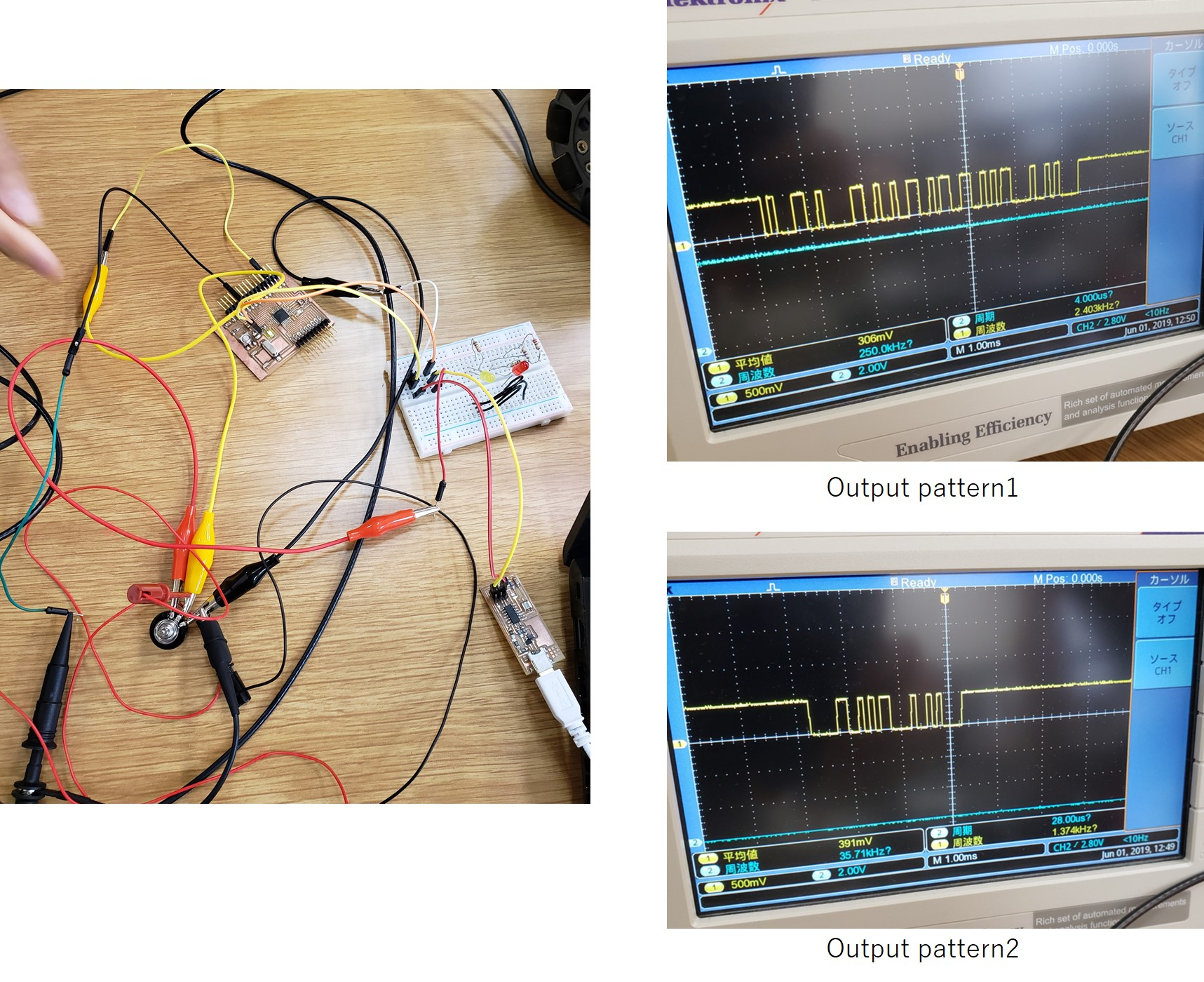# 11. Input devices¶

The group assignment this week was probe an input device’s analog levels and digital signals.

## Potentiometer¶

For this assignment, we used a potentiometer. Potentiometers can change the values of its resistors by rotating (variable resistor). As below figure of electrical circuit shows, when the resistor changes, the voltage between terminal1 and 2 also changes, so reading the voltage means changed resistors.Actual wiring and output patterns are shown below figure. For this assignment, Satshakit was used instead of Arduino. Two output patterns compare with maximum and minimum changed variables.In the figure, the yellow waves mean analog level and the blue waves show digital level. From the result of measuring, when the variable resistor of the potentiometer changed, both digital and analog level changed.